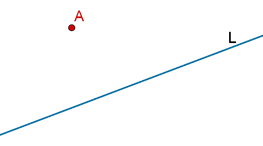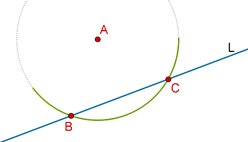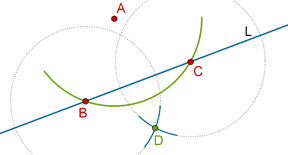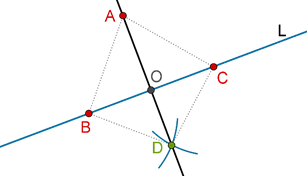# Constructing Perpendicular from Point to Line

Go back to  'Triangles-Quadrilaterals'

Suppose that you are given a line L, and a point A not on L:How can you construct the perpendicular to L through A?

Step 1: Taking A as center and a sufficiently large radius, draw an arc which intersects L at two points, B and C, as shown below:Can you see why the radius of this arc must be greater than a minimum value? What is that minimum value?

Step 2: Taking B and C as centers and a radius equal to more than ½ BC, draw two arcs which intersect each other at D:Step 3: Draw a line through A and D. This is the required perpendicular.Proof: Compare $$\Delta ABD$$ and $$\Delta ACD$$:

1. AB = AC (radii of the same circular arc)

2. BD = CD (arcs of the same radii)

By the SSS criterion, the two triangles are congruent, which means that $$\angle BAO$$ = $$\angle CAO$$. Now, compare $$\Delta BAO$$ and $$\Delta CAO$$:
2. $$\angle BAO$$ = $$\angle CAO$$ (shown above)
$$\angle BOA$$ = $$\angle COA$$ = ½ (1800) = 900